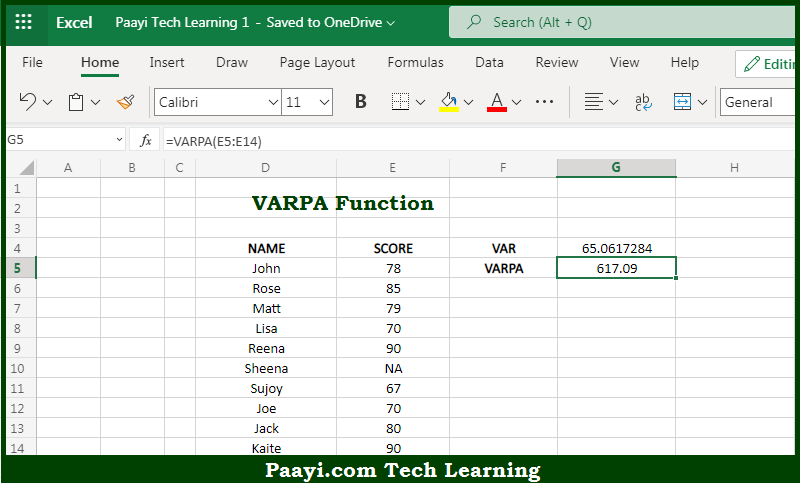# Learn How to Use Microsoft Excel VARPA Function

Written by | 0 Comments | 449 Views

In this article, you will learn how to use the Microsoft Excel VARPA function and its prime function in Microsoft Excel. You will also get to know the Microsoft Excel VARPA function return value and syntax with the help of some examples.

Microsoft Excel VARPA Function

The main purpose of the Microsoft Excel VARPA function is to get the variation of the sample or population. That implies, with the help of the VARPA function you can able to calculate the variance of the sample of a given data set or population. It should be noted that the VARPA function evaluates text values and the logical in references, unlike the VARP function. So, with the help of the VARPA function, you can able to get the variation of the entire sample or population.

Return Value of VARPA Function

The return value will be the calculated variance.

Syntax of VARPA Function

=VARPA(number1, [number2], ...)

Where the arguments:

• number1: This is the first number or reference.
• number2: This is the second number or reference (optional).

## How to Use Microsoft Excel VARPA Function?So we know that Microsoft Excel VARPA function you can able to get the variation of the sample or population. That implies, with the help of the VARPA function you can able to calculate the variance of the sample of a given data set or population. It should be noted that the VARPA function evaluates text values and logical in references, unlike the VARP function. So, with the help of the VARPA function, you can able to get the variation of the entire sample or population.